# NCERT Solutions: Factorisation- 1 Notes | Study Mathematics (Maths) Class 8 - Class 8

## Class 8: NCERT Solutions: Factorisation- 1 Notes | Study Mathematics (Maths) Class 8 - Class 8

The document NCERT Solutions: Factorisation- 1 Notes | Study Mathematics (Maths) Class 8 - Class 8 is a part of the Class 8 Course Mathematics (Maths) Class 8.
All you need of Class 8 at this link: Class 8

Question: Factorise
(i) 12x + 36
(ii) 22y – 33z
(iii) 14pq + 35pqr
Solution:
(i) We have 12x = 3 * 2 * 2 * x = (2 * 2 * 3) * x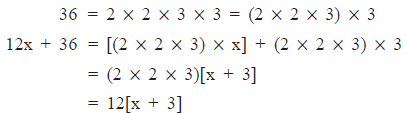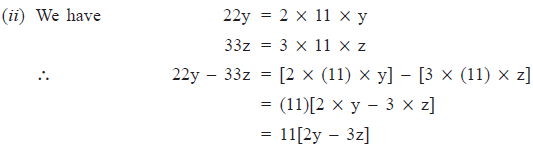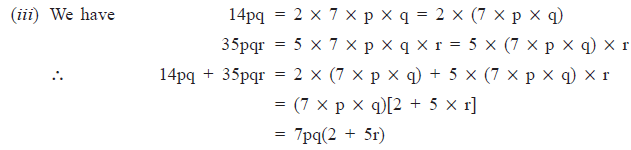Exercise 14.1

Question 1. Find the common factors of the given terms.
(i) 12x, 36
(ii) 2y, 22xy
(iii) 14pq, 28p2q2
(iv) 2x, 3x2, 4
(v) 6abc, 24ab2, 12a2b
(vi) 16x3, –4x2, 32x
(vii) 10pq, 20qr, 30rp
(viii) 3x2y3, 10x3y2, 6x2y2xz

Solution: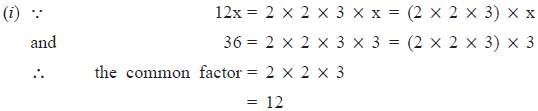(ii) ∵                         2y = 2 * y = (2 * y)
and                        22y = 2 * 11 X y = (2 X y) X 11
∴ the common factor   = 2 X y
= 2y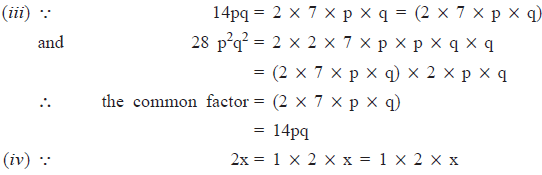3x2 = 1 * 3 * x * x = 1 * 3 * x
and                                  4 = 1 * 2 * 2 = 1 * 2 * 2
∴ the common factor = 1
Note: 1 is a factor of every term.

(v) ∵            6abc = 2 * 3 * a * b * c = (2 * 3 * a * b) * c
24 ab2 = 2 * 2 * 2 * 3 * a * b * b = (2 * 3 * a * b) * 2 * 2 * b
12 a2b = 2 * 2 * 3 * a * a * b = (2 * 3 * a * b) * 2 * a
∴ the common factor = 2 * 3 * a * b
= 6ab

(vi) ∵                   16x3 = 2 * 2 * 2 * 2 * x * x * x = (2 * x * x
–4x2 = –1 * 2 * 2 * x * x = (2 * 2 * x) * (–1) * x
32x = 2 * 2 * 2 * 2 * 2 * x = (2 * 2 * x) * 2 * 2 * 2
∴ the common factor = 2 * 2 * x
= 4x

(vii) ∵                10pq  = 2 * 5 * p * q = (2 * 5) * p * q
20qr = 2 * 2 * 5 * q * r = (2 * 5) * 2 * q * r
30rp = 2 * 3 * 5 * r * p = (2 * 5) * 3 * r * p
∴ the common factor  = 2 * 5
= 10

(viii) ∵                3x2y3 = 3 * x * x * y * y * y
= (x * x * y * y) * 3 * y
10x3y2 = 2 * 5 * x * x * x * y * y
= (x * x * y * y) * 2 * 5 * x
6x2y2z = 2 * 3 * x * x * y * y * z
= (x * x * y * y) * 2 * 3 * z
∴ the common factor   = (x * x * y * y)
= x2y2

Question 2. Factorise the following expressions.
(i) 7x – 42
(ii) 6p – 12q
(iii) 7a2  + 14a
(iv) –16z + 20z3 (v) 20 l2m + 30alm
(vi) 5x2y – 15xy2
(vii) 10a2 – 15 b2 + 20c2
(viii) –4a2 + 4ab – 4ca
(ix) x2yz + xy2z + xyz2
(x) ax2y + bxy2 + cxyz

Solution:
(i) ∵           7x = 7 * x = (7) * x
42 = 2 * 7 * 3 = (7) * 2 * 3
∴                        7x – 42 = 7[(x) – (2 * 3)]
= 7[x – 6]

(ii) ∵          6p = 2 * 3 * p = (2) * (3) * p = (2 * 3) * p
12q = 2 * 2 * 3 * q = (2) * 2 * (3) * q = (2 * 3) * 2 * q
∴     6p – 12q = (2 * 3) [(p) – (2 * q)]
= 6[p – 2q]

(iii) ∵           7a2 = 7 * a * a = (7 * a) * a
14a = 2 * 7 * a = (7 * a) * 2
∴       7a2 – 14a = (7 * a)[a + 2]
= 7a(a + 2)

(iv) ∵       –16z = (–1) * 2 * 2 * 2 * 2 * z = (2 * 2 * z) * (–1) * 2
20z3 = 2 * 2 * 5 * z * z * z = (2 * 2 * z) * 5 * z * z
∴  –16z + 20z3 = (2 * 2 * z) [(–1) * 4 + 5 * z * z]
= 4z[–4 + 5z2]

(v) ∵         20l2m = 2 * 2 * 5 * l * l * m = (2 * 5 * l * m) * 2 * l
30alm = 2 * 3 * 5 * a * l * m = (2 * 5 * l * m) * 3 * a
∴ 20l2m + 30alm = (2 * 5 * l * m)[ 2 * l + 3 * a]
= 10lm[2l + 3a]

(vi) ∵           5x2y = 5 * x * x * y = (5 * x * y)[x]
15xy2 = 5 * 3 * x * y * y = (5 * x * y)[3 * y]
∴    5x2y – 15xy2 = (5 * x * y)[x – 3 * y]
= 5xy(x – 3y)

(vii) ∵                10a2 = 2 * 5 * a * a = (5)[2 * a * a]
15b2 = 3 * 5 * b * b = (5)[3 * b * b]
20c2 = 2 * 2 * 5 c * c = (5)[2 * 2 * c * c]
∴ 10a2 – 15b2 + 20c2 = (5)[2 * a * a + 3b * b + 2 * 2 * c * c]
= 5[2a2 + 3b2 + 4c2]

(viii) ∵              –4a2 = (–1) * 2 * 2 * a * a = (2 * 2 * a)[(–1) * a]
4ab = 2 * 2 * a * b = (2 * 2 * a)[b]
–4ca = (–1) * 2 * 2 * c * a = (2 * 2 * a)[(–1) * c]
∴ –4a2 + 4ab – 4ca = (2 * 2 * a)[(–1) * a + b – c]
= 4a[–a + b –c]

(ix) ∵                  x2yz = x * x * y * z = (xyz)[x]
xy2z = x * y * y * z = (xyz)[y]
xyz2 = x * y * z * z = (xyz)[z]
∴ x2yz + xy2z + xyz2 = (xyz)[x + y + z]
= xyz(x + y + z)

(x) ∵                  ax2y = a * x * x * y = (x * y)[a * x]
bxy2 = b * x * y * y = (x * y)[b * y]
cxyz = c * x * y * z = (x * y)[c * z]
∴ ax2y + bxy2 + cxyz = (x * y)[a * x + b * y + c * z]
= xy(ax + by + cz)

Question 3. Factorise:
(i) x2 + xy + 8x + 8y
(ii) 15xy – 6x + 5y – 2
(iii) ax + bx – ay – by
(iv) 15pq + 15 + 9q + 25p
(v) z – 7 + 7xy – xyz
Solution:

(i) x2 + xy + 8x + 8y = x[x + y] + 8[x + y]

= (x + y)(x + 8)

(ii) 15xy – 6x + 5y – 2 = 3x[5y – 2] + 1[5y – 2]

= (5y – 2)(3x +1)

(iii) ax + bx – ay – by = x[a + b] + (–y)[a + b]

= (x – y)[a + b]

(iv) Regrouping the terms, we have

15pq + 15 + 9q + 25p = 15pq + 9q + 25 p + 15

= 3q[5p + 3] + 5[5p + 3]

= (5p + 3)[3q + 5]

(v) Regrouping the terms, we have

z – 7 + 7xy – xyz = z – 7 – xyz + 7xy

= 1[z – 7] – xy[z – 7]

= (z – 7)(1 – xy)

Factorisation Using Identities

We know that

(a + b)2 = a2 + 2ab + b2

(a – b)2 = a2 – 2ab + b2

(a + b)(a – b) = a2 – b2

Using these identities, we can say that

a2 + 2ab + b2 = (a + b)2

= (a + b)(a + b)

a2 – 2ab + b2 = (a – b)2

= (a – b)(a – b)

a2 – b2 = (a + b)(a – b)

Note: For factorising an expression of the type x2 + px + q [where p = (a + b) and q = (ab)], we have

x2 + px + q = x2 + (a + b)x + ab

= x2 + ax + bx + ab

= x(x + a) + b(x + a)

= (x + a)(x + b)

Exercise 14.2
Question 1. Factorise the following expressions.

(i) a2 + 8a + 16

(ii) P2 – 10p + 25

(iii) 25m2 + 30 m + 9

(iv) 49y2 + 84yz + 36z2

(v) 4x2 – 8x + 4

(vi) 121b2 – 88bc + 16c2

(vii) (l + m)2 – 4lm

(viii) a4 + 2a2b2 + b4

[Hint: Expand (l + m)2 first.]

Solution:

(i) We have   a2 + 8a + 16 = (a)2 + 2(a)(4) + (4)2

= (a + 4)2 = (a + 4)(a + 4)

∴                   a2 + 8a + 16 = (a + 4)2 = (a + 4)(a + 4)

(ii) We have p2 – 10p + 25 = P2 – 2(p)(5) + (5)2

= (p – 5)2 = (p – 5)(p – 5)

∴                  P2 – 10p + 25 = (p – 5)2 = (p – 5)(p – 5)

(iii) We have     25m2 + 30m + 9 = (5m)2 + 2(5m)(3) + (3)2

= (5m + 3)2 = (5m + 3)(5m + 3)

∴                       25m2 + 30m + 9 = (5m + 3)2 = (5m + 3)(5m + 3)

(iv) We have 49y2 + 84yz + 36z = (7y)2 + 2(7y)(6z) + (6z)2

= (7y + 6z)2

= (7y + 6z)(7y + 6z)

∴                   49y2 + 84yx + 36 z2 = (7y + 6z)2 = (7y + 6z)(7y + 6z)

(v) We have 4x2 – 8x + 4 = (2x)2 – 2(2x)(2) + (2)2

= (2x – 2)2 = (2x – 2)(2x – 2)

= 2(x – 1)2(x – 1)

= 4(x – 1)(x – 1)

∴                 4x2 – 8x + 4 = 4(x – 1)(x – 1) = (4(x – 1)2

(vi) We have 121b2 – 88bc + 16c2 = (11b)2 – 2(11b)(4c) + (4c)2

= (11b – 4c)2 = (11b – 4c)(11b – 4c)

∴                  121b2 – 88bc + 16c2 = (11b – 4c)2 = (11b – 4c)(11b – 4c)

(vii) We have   (l + m)2 – 4lm = (l2 + 2lm + m2) – 4lm

[Collecting the like terms 2lm and –4lm]

= l2 + (2lm – 4lm) + m2

= l2 + 2lm + m2

= (l)2 – 2(l)(m) + (m)2

= (l – m)2 = (l – m)(l – m)

∴                  (l + m)2 – 4lm = (l – m)2 = (l – m)(l – m)

(viii) We have a4 + 2a2b2 + b4 = (a2)2 + 2(a2)(b2) + (b2)2

= (a2 + b2)2 = (a2 + b2)(a2 + b2)

∴                   a4 + 2a2b2 + b2 = (a2 + b2)2 = (a2 + b2)(a2 + b2)

Question 2. Factorise:

(i) 4p2 – 9q2

(ii) 63a2 – 112b2

(iii) 49x2 – 36

(iv) 16x5 – 144x3

(v) (l + m)2 – (l – m)2

(vi) 9x2y2 – 16

(vii) (x2 – 2xy + y2) – z2

(viii) 25a2 – 4b2 + 28bc – 49c2

Solution:

(i) ∵ 4p2 – 9q2 = (2p)2 – (3q)2

= (2p – 3q)(2p + 3q)        [Using a2 – b2 = (a + b)(a – b)]

∴    4p2 – 9q2 = (2p – 3q)(2p + 3q)

(ii) We have

63a2 – 112b2 = 7 * 9a2 – 7 * 16b2

= 7[9a2 – 16b2)

∵       9a2 – 16b2 = (3a)2 – (4b)2

= (3a + 4b)(3a – 4b)          [Using a2 – b2 = (a + b)(a – b)]

∴ 63a2 – 112b2 = 7[(3a + 4b)(3a – 4b)]

(iii) ∵  49 x2 – 36 = (7x)2 – (6)2

= (7x – 6)(7x + 6)              [Using a2 – b2 = (a – b)(a + b)]

∴        49x2 – 36 = (7x – 6)(7x + 6)

(iv) We have 16x5 = 16x3 * x2 and 144x3 = 16x3 * 9

∴     16x5 – 144x3 = (16x3) * x2 – (16x3) * 9

= 16x3[x2 – 9]

= 16x3[(x)2 – (3)2]

= 16x3[(x + 3)(x – 3)]          [(Using a2 – b2 = (a – b)(a + b)]

∴     16x5 – 144x3 = 16x3(x + 3)(x – 3)

(v) Using the identitiy a2 – b2 = (a + b)(a – b), we have

(l + m)2 – (l – m)2 = [(l + m) + (l – m)][(l + m) – (l – m)]

= [l + m + l – m][l + m – l + m]

= (2l)(2m) = 2 * 2(l * m)

= 4lm

(vi) We have    9x2y2 – 16 = (3xy)2 – (4)2

= (3xy + 4)(3xy – 4)        [Using a2 – b2 = (a + b)(a – b)]

∴                      9x2y2 – 16 = (3xy + 4)(3xy – 4)

(vii) We have x2 – 2xy + y2= (x – y)2

∴         (x2 – 2xy + y2) – z2 = (x – y)2 – (z)2

= [(x – y) + z][(x – y) – z]

[Using a2 – b2 = (a + b)(a – b)]

= (x – y + z)(x – y – z)

∴       (x2 – 2xy + y2) – z2 = (x – y + z)(x – y – z)

(viii) We have       –4b2 + 28bc – 49c2 = (–1)[4b2 – 28bc + 49c2]

= –1[(2b)2 – 2(2b)(7c) + (7c)2]

= –[2b – 7c]2

∴                25a2 – 4b2 + 28bc – 49c2  = 25a2 – (2b – 7c)2

Now, using                              a2 – b2 = (a + b)(a – b), we have

[5a]2 – [2b – 7c]2 = (5a + 2b – 7c)(5a – 2b + 7c)

∴               25a2 – 4a2 + 28bc – 49c2 = (5a + 2b – 7c)(5a – 2b + 7c)

Question 3. Factorise the expressions.
(i) ax2 + bx
(ii) 7p2 + 21q2
(iii) 2x3 + 2xy2 + 2xz2
(iv) am2 + bm2 + bn2 + an2
(v) (lm + l) + m + 1
(vi) y(y + z) + 9(y + z)
(vii) 5y2 – 20y – 8z + 2yz
(viii) 10ab + 4a + 5b + 2
(ix) 6xy – 4y + 6 – 9x
Solution:
(i)
∵       ax2 = a * x * x = (x)[ax]

bx = b * x = (x)[b]

∴   ax2 + bx = x[ax + b]

(ii) We have 7p2 + 21q2 = 7 * p * p + 7 * 3 * q * q

= 7[p * p + 3 * q * q]

= 7[p2 + 3q2]

(iii) Taking out 2x as common from each term, we have

2x3 + 2xy2 + 2xz2 = 2x[x2 + y2 + z2]

(iv) We can take out m2 as common from the first two terms and n2 as common from the last two terms,

∴ am2 + bm2 + bn2 + an2 = m2(a + b) + n2(b + a)

= m2(a + b) + n2(a + b)

= (a + b)[m2 + n2]

(v) We have     lm + l = l(m + 1)

∴    (lm + l) + (m + 1) = l(m + 1) + (m + 1)

= (m + 1)[l + 1]

(vi) We have (y + z) as a common factor to both terms.

∴     y(y + z) + 9(y + z) = (y + z)(y + 9)

(vii) We have 5y2 – 20y = 5y(y – 4)

and                –8z + 2yz = 2z(–4 + y)

= 2z(y – 4)

∴ 5y2 – 20y – 8z + 2yz = 5y(y – 4) + 2z(y – 4)

= (y – 4)[5y + 2z]

(viii) We have,    10ab + 4a = 2a(5b + 2)

and                         (5b + 2) = 1(5b + 2)

∴           10ab + 4a + 5b + 2 = 2a(5b + 2) + 1(5b + 2)

= (5b + 2)[2a + 1]

(ix) Regrouping the terms, we have

6xy – 4y + 6 – 9x = 6xy – 4y – 9x + 6

= 2y[3x – 2] – 3[3x – 2]

= (3x – 2)[2y – 3]

Question 4. Factorise:
(i) a4 – b4
(ii) p4 – 81
(iii) x4 – (y + z)4
(iv) x4 – (x – z)4
(v) a4 – 2a2b2 + b4
Solution:
(i)
Using a2 – b2 = (a – b)(a + b), we have

a4 – b4 = (a2)2 – (b2)2

= (a2 + b2)(a2 – b2)

= (a2 + b2)[(a + b)(a – b)]

= (a2 + b2)(a + b)(a – b)

(ii) We have    P4 – 81 = (p2)2 – (92)2

Now using      a2 – b2 = (a + b)(a – b), we have

(p2)2 – (9)2 = (p2 + 9)(p2 – 9)

We can factorise p2 – 9 further as

p2 – 9 = (p)2 – (3)2

= (p + 3)(p – 3)

∴                  p4 – 81 = (p + 3)(p – 3)(p2 + 9)

(iii)  ∵ x4 – (y + z)4 = [x2]2 – [(y + z)2]2

= [(x)2 + (y + z)2][(x2) – (y + z)2]

[Using a2 – b2 = (a + b)(a – b)]

We can factorise [x2 – (y + z)2] further as

(x)2 – (y + z)2 = [(x) + (y + z)][(x) – (y + z)]

= (x + y + z)(x – y – z)

∴       x4 – (y + z)4 = (x + y + z)(x – y – z)[x2 + (y + z)2]

(iv) We have x4 – (x – z)4 = [x2]2 – [(x – z)2]2

= [x2 + (x – z)2][x2 – (x – z)2]

Now, factorising x2 – (x – z)2 further, we have

x2 – (x – z)2 = [x + (x – z)][x – (x – z)]

= (x + x – z)(x – x + z) = (2x – z)(z)

∴                x4 – (y – z)4 = z(2x – z)[x2 + (x – z)2]

= z(2x – z)[x2 + (x2 – 2xz + z2)]

= z(2x – z)[x2 + x2 – 2xz + z2]

= z(2x – z)(2x2 – 2xz + z2)

(v) ∵   a4 – 2a2b2 + b4 = (a2)2 – 2(a2)(b2) + (b2)2

= (a2 – b2)2

= [(a2 – b2)(a2 + b2)]

= [(a – b)(a + b)(a2 + b2)]

∴         a4 – 2a2b2 + b4 = (a – b)(a + b)(a2 + b2)

Question 5. Factorise the following expressions.
(i) p2 + 6p + 8
(ii) q2 – 10q + 21
(iii) p2 + 6p – 16
Solution:
(i)
We have     p2 + 6p + 8 = P2 + 6p + 9 – 1                [∵ 8 = 9 – 1]

= [(p)2 + 2(p)(3) + 32] – 1

= (p + 3)2 – 12      [Using a2 + 2ab + b2 = (a + b)2]

= [(p + 3) + 1][(p + 3) – 1]

= (p + 4)(p + 2)

∴                     p2 + 6p + 8 = (p + 4)(p + 2)

(ii) We have     q2 – 10q + 21 = q2 – 10q + 25 – 4       [∵ 21 = 25 – 4]

= [(q)2 – 2(q)(5) + (5)2] – (2)2

= [q – 5]2 – 2

= [(q – 5) + 2][(q – 5) – 2]

[Using a2 – b2 = (a + b) (a – b)]

= (q – 3)(q – 7)

(iii) We have      p2 + 6p – 16 = p2 + 6p + 9 – 25          [∵ –16 = 9 – 25]

= [(p)2 + 2(p)(3) + (3)2] – (5)2

= [p + 3]2 – 2 [Using a2 + 2ab + b2 = (a + b)2]

= [p + 3] + 5][(p + 3) – 5]

[Using a2 – b2 = (a + b)(a – b)

= (p + 8)(p – 2)

Division of Algebraic Expressions

For division of algebraic expression by another expression:

1. We write them in the form of a fraction, such that the divisor is the denominator.
2. Factorise the numerator as well as the denominator.
3. Cancel the factors common to both the numerator and denominator.

Example 1. Divide 33(p4 + 5p3 – 24p2) by 11p(p + 8).
Solution: We have 33(p4 + 5p3 – 24p2) ÷ 11p(p + 8)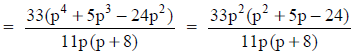[In numerator, p is taken out common from each term]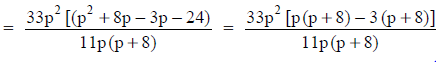[In numerator, 5p is splitted in to 8p – 3p such that (8p)(–3p) = –24p]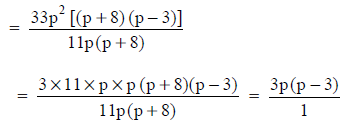[Cancelling the factors 11p and x + 8 common to both the numerator and denominator]
Thus, 33(p4 + 5p3 – 24p2) ÷ 11p(p + 8)
= 3p(p – 3)

The document NCERT Solutions: Factorisation- 1 Notes | Study Mathematics (Maths) Class 8 - Class 8 is a part of the Class 8 Course Mathematics (Maths) Class 8.
All you need of Class 8 at this link: Class 8Use Code STAYHOME200 and get INR 200 additional OFF

## Mathematics (Maths) Class 8

138 videos|321 docs|48 tests

Track your progress, build streaks, highlight & save important lessons and more!

,

,

,

,

,

,

,

,

,

,

,

,

,

,

,

,

,

,

,

,

,

;